Journal of Creation 19(1):82–87, April 2005

# Dark matter and a cosmological constant in a creationist cosmology?

Using the centro-symmetric cosmology of Moshe Carmeli, it is shown that there is no need to assume the existence of dark matter to explain dynamics of galaxies in the cosmos. Further, it is shown that in this cosmology the cosmological constant or dark energy is a property of space-time. This can be interpreted in a creationist cosmology as the power of the Lord giving a boost to the expansion of the fabric of space as He stretched it out. He is the unseen force in the universe. By the correct choice of field equations, the motions of the galaxies are described without the need to resort to exotic particles. This description fits a finite galactocentric universe, and is consistent with a creationist cosmology.

Dark matter is the term for the hypothesized matter in the universe required to explain the missing mass problem of the standard cosmological / big bang model. Dark matter supposedly interacts with normal matter by gravity, but does not absorb or emit radiation, and thus cannot be seen. Big bang cosmologists propose that about 25% of the universe is made up of dark matter (possibly consisting of non-standard particles, such as neutrinos, axions or weakly interacting massive particles [WIMPs]).1 70% of the universe in their models is made up of the even more obscure dark energy, leaving 5% of the universe as ordinary matter.

In the nineteenth century, dark matter was once blamed for the anomalous advance of Mercury’s perihelion.2 Mercury’s elliptical orbit around the sun advances, or precesses, by a very small amount each orbit. The expected precession, according to Newtonian and Keplarian laws of planetary motion, was inexplicably exceeded by 43 seconds of arc per century.

If our solar system was comprised only of the sun and one planet, that planet would retrace its elliptical path perfectly forever, assuming Newton’ Law of Gravity was all there was. The presence of other planets destroys this perfection because of the small gravitational forces they exert on each other. However, those effects are completely predictable. The anomalous effect on Mercury’s orbit, described above, was not predictable by any known theories of gravitation at the time.

Figure 1. Plot of equation (1) with Ωm = 1 and 0.24 and equation (4) with Ω0 = 0.03. Notice, the top two curves lay on top of each other. The broken curve represents a free, or coasting, expansion where Ωm = 1 over all redshifts.

One possible explanation was that there might be an undetected planet even closer to the sun than Mercury itself. (Neptune, for example, had been predicted and discovered quite easily, a success which seemed to confirm Newtonian gravity in every respect.) The hypothetical planet was appropriately named Vulcan, after the Roman god of fire, since it was believed to lie very close to the hot sun. But, alas, no such planet was ever found, though there were claims and counterclaims.

In 1915 Einstein solved the problem with the publication of his General Theory of Relativity. It showed that the anomalous precession is a consequence of the way gravity distorts space and time, and controls the motions of planets when they get particularly close to massive bodies, where the curvature of space is most pronounced. Newtonian gravitation is not an accurate enough description of planetary motion when space curvature departs from Euclidean flatness; General Relativity explains the observed behaviour almost exactly.

So neither dark matter, which some conjectured to be in an unobserved ring of matter around the sun, nor the planet Vulcan itself was necessary to explain the anomaly. Neither was any unobservable exotic material needed. This brings to mind what the Bible says:

‘For the invisible things of him from the creation of the world are clearly seen, being understood by the things that are made, even his eternal power and Godhead; so that they are without excuse’ (Rom. 1:20, KJV).
‘And I set my heart to seek and search out by wisdom concerning all that is done under heaven; this burdensome task God has given to the sons of man, by which they may be exercised’ (Eccl. 1:13, NKJV).

In the natural realm it seems that the Lord has designed His laws so that they can be understood in terms of what we can observe. We don’t need to conceive of exotic, undetectable material in order to make this work; rather, by starting with the revelationary wisdom of His Word, we have a starting point for searching out the solution.

## Einstein’s cosmological constant, Λ

From his General Theory of Relativity, Einstein constructed a cosmological explanation of the universe, based on a 4-dimensional space-time metric. He saw that within this model the universe would tend to collapse under gravitation so he added a constant (represented by the Greek symbol Λ—lambda) to his field equations to maintain a static universe. Its value was extremely small, yet on the scale of the universe it had the effect of pushing the galaxies apart.

This model was developed before Einstein heard of the observations of Edwin Hubble that indicated the galaxies in the universe were (apparently) speeding away from us; i.e. the universe was expanding. Einstein immediately dropped the parameter, supposedly saying that it was the biggest blunder of his life.

Figure 2. The parameters ΩΛ and Ωm as functions of redshift, z

In recent years cosmologists have reinvoked the cosmological constant in big bang inflationary cosmologies, primarily because astronomers looking at high-redshift supernovae claim the universe is accelerating.3-7 This acceleration is only observed at very high redshifts (z > 0.5), where the data could also be made to fit a wide range of other parameters.8 Since this discovery of acceleration, astronomers have started to speak of dark matter again, and the new concept of dark energy.

I contend that dark matter doesn’t exist. It is simply, as before, the result of insufficient understanding of God’s laws at work. There are many cosmological descriptions (cosmological metrics) to the universe; if the wrong model is applied, cosmological thinking will head in the wrong direction. I believe that the standard Friedmann–Lemaître (FL) model is an incorrect description because of its starting assumption of no centre to the universe.9 Furthermore, modern cosmologists have failed to recognize the hand of God in the expansion of the universe.

This paper analyzes the creationist centro-symmetric universe in terms of Carmeli’s cosmological construction.10 Using this we can explain the accelerating universe, without dark matter, but with a term called dark (i.e. not visible to us) energy, which is the result of God’s action during the early part of the creation, on Day 4. No cosmological constant is needed when the correct field equations are chosen in this model. In fact, this means even the dark energy term is really only an effective term as it really is a property of the correctly chosen equations of motion of the heavenly bodies in this new cosmology.

The Carmeli model is certainly non-standard cosmology and has not been accepted by the adherents to the standard paradigm.11 For an introduction to the basic equations, see section C of ‘A creationist cosmology in a galactocentric universe’,12 as well as references 10 and 13. Using his interpretation of Einstein’s field equations, Carmeli’s cosmological model predicted the form of the high-z14 supernovae measurements,3-7 data that indicates the universe is not only expanding but also accelerating. To do this, he assumed a value of dark matter density for his model.

I will show that if he had instead assumed that the density of normal matter was not fixed, but depends on the distance we look back into the cosmos (an assumption based on the fact that the universe was smaller in the past), then he would have found he didn’t need to assume the existence of any dark matter at all.15

## Redshift distance relation

If we rewrite equation (22c) from ‘A creationist cosmology in a galactocentric universe’,12 in terms of natural units and for redshift (z) but arbitrary density (Ωm), which is the averaged matter density of the universe expressed as a fraction of the ‘closure’ density, it becomes: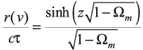(1)

where r is the radial distance to the galaxy and c is the speed of light.16 The parameter

is the time constant of the universe, a constant at any epoch and approximately the reciprocal of the Hubble constant. It is related to a new constant in Carmeli’s model h = 1/ , which is not redshift dependent.

Equation (1) is the Hubble relation, which, when taken for small redshift (z), reduces to the Hubble Law. For small z the right-hand side (rhs) of (1) is approximately z and the left-hand side (lhs) becomes H0/c. Expanding the rhs to the second term (using a power law approximation for sinh) yields the equation used by Carmeli to predict the form of the high-z supernovae measurements.17 (Equation 5.21 of ref. 18, which is reproduced here.)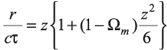where Ωm<1 (2)

Calculations show that (1) and (2) are nearly identical for z < 1. To make his equation fit the high-z supernovae measurement data, Carmeli assumed a value of matter density of Ωm = 0.245, which was the accepted value in 1998, and corresponds to the presently accepted Ωm = 0.3 in the FL cosmologies. This density is assumed to be made up of mostly dark matter.

But let us instead consider what happens to the density of matter as we look back in time, when the universe was smaller. (When we look out into the cosmos at redshifted light, we are looking backwards in time.) Carmeli assumed that the value of Ωm was fixed in his curve fitting. However, Ωm varies as a function of z. For flat space it is normally assumed: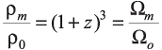(3)

where Ρm(z) is the averaged matter density of the universe at the redshift value z, and Ρ0 is the averaged matter density of the universe locally or near z = 0. The parameter Ω0 is then the locally averaged matter density of the universe expressed as a fraction of the critical density. Here we assume only normal baryonic atomic matter. Equation (3) results from the fact that as the redshift increases, the volume decreases as (1 + z)3. Notice that at z = 1 the universe is 8 times smaller in volume and therefore it is 8 times denser. That is, at z = 1, Ωm = 8 Ω0.

Substituting (3) into (1) we get: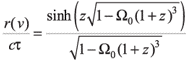(4)

Following the same approach as Carmeli, I have plotted (in figure 1) Carmeli’s equation (1) with Ωm = 0.24 and my equation (4) with Ω0 = 0.03, which is within the bounds of the locally measured (z ≈ 0) value for baryonic matter.19 Comparing the two equations between z = 0.25 and z = 1, which is the critical domain of the analysis, we get the following pairs of values for

shown in table 1.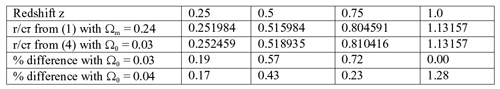Table 1. Comparison of redshift distance equations

From table 1 it can be seen that the difference between the two equations over the domain of the measurements is much less significant than the fit to the data. The supernovae distances measured were 10%–15% farther out than expected and many points don’t touch the curve fit within 1 σ error bars. If we assume Ω0 = 0.04 instead of Ω0 = 0.03, both of which are within measured parameters, we get closer agreement at smaller redshift but a poorer fit near z = 1.

Figure 3. The parameter ΩΛ plotted as a function of Ωm. Note: the axis for Ωm has been reversed, running from large to small.

From table 1 it is seen that a local matter density of only Ω0 = 0.03—0.04 is necessary to have good agreement with observation in the local part of the universe. This, then, effectively eliminates the need for dark matter.

Using equation (24) from ‘A creationist cosmology in a galactocentric universe’,12 which is approximated for small z, Carmeli gives a value for h = 80 km s-1 Mpc. This gives the time constant

= h-1 = 3.85 ×1017 s at this current epoch.

## Dark energy

Carmeli’s cosmological model assumes20 that the expansion of the universe starts with Ωm > 1 and uses equation (1) to describe the expansion. The early expansion involves a deceleration followed by a point where Ωm = 1 (coasting) and then an accelerating expansion with Ωm < 1.

The FL model as sumes a homogeneous, isotropic universe. In order that the expansion of the universe accelerates, the FL inflation cosmologies21 have had to return the cosmological constant, Λ, to Einstein’s field equations to become: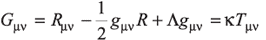(5)

By comparing his model with the standard FL model, Carmeli was able to determine a value for the contribution of Λ to the mass/energy density of the universe, though it does not explicitly appear in the Carmeli cosmology. This parameter ΩΛ has often been called the contribution from the vacuum energy density and given the parameter ΩΛ, when expressed as a fraction of the critical, or ‘closure’, density. The critical density in the FL models is

, while in Carmeli’s model ρc = 3h2/8πG. Thus, Carmeli showed22 that ΩΛ = (H0/h)2.

The WMAP value of H0 gives a value of ΩΛ = 0.782 (at z = 1), where I have used the form of (4) with Ω0 = 0.03. Carmeli produced a similar result of ΩΛ = 0.764 when he used (24) from ‘A creationist cosmology in a galactocentric universe’ and Ωm = 0.245. 23 Hence, Carmeli showed ΩΛ + Ωm= 1.009 and reported that space was essentially flat-Euclidean geometry (see figure 4).

Taking this further, we get, for the fraction of dark energy,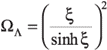where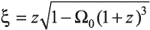(6)

Dark energy is also referred to as vacuum energy and (6) describes the evolution of the fraction of this vacuum energy (ΩΛ) as a function of redshift (z). This parameter ΩΛ represents a force that pushes the galaxies outward, away from each other. In Carmeli’s model the pressure

kg m-2 is positive, where Ωm < 1. It is not negative as is required in FL and Gentry’s cosmologies.12

In FL cosmologies dark energy is interpreted as either vacuum energy (cosmological constant) or as the slowly changing energy of a scalar field with a vacuum-like equation of state p = w ρV, where the parameter is model-dependent but usually w = –1. WMAP data indicates w = –0.78.24 This is where Gentry gets his negative mass term.

Remember, neither ΩΛ nor Λ appear explicitly in Carmeli’s model. It is only by a comparison with FL models that the assignment can be made. This means that dark energy is really a property of space-time, or more correctly space-velocity as Carmeli calls it. By writing ΩΛ as a function of z we can get an idea of its behaviour over time (figure 2).

As we look back in time in the cosmos the matter density increases according to (1 + z)3. So we see the effect on ΩΛ, though (6) may not be valid for Ωm + ΩΛ >> 1 because of the assumption in (3). Equation (7), however, should remain valid, although we don’t know how the density varies at high redshift. Figure 2 shows both the values of ΩΛ, Ωm and ΩΛ + Ωm as a function of redshift, z. The parameter ΩΛ starts at the origin with the value of unity and as z increases and density increases, ΩΛ initially decreases but then starts to grow rapidly past z = 1.5. At a redshift of z = 1 this model yields ΩΛ = 0.78, Ωm = 0.24 and ΩΛ + Ωm = 1.02.

Obviously, this analysis is still limited by the assumptions in (3) but if we eliminate z from (6) by writing

we get:25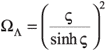where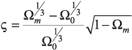(7)

In figure 3, I have plotted ΩΛ as a function of Ωm from (7). It indicates that as the mass/energy density increases, ΩΛ becomes extremely large. This means as we look back into the past towards the creation at the beginning of Day 4, when God stretched out the heavens, we see a very large cosmological constant contribution or vacuum energy density, which decreases with time running forward. This may be interpreted to mean that God gave the expansion a big boost at the beginning to overcome the initial tendency for matter to collapse on itself instead of expanding. It is important to reiterate that these effects are properties of the correct field equations, which I see are the descriptions of God’s actions and laws in the universe.

From (6) and (7) it follows that as the universe expands the total density tends to a vacuum energy density ΩΛ = 1 (since Ω0 = 0). This means a totally spatially flat universe in a totally relaxed state. In figure 3 as Ωm →  0 we see ΩΛ → 1, but in the form of a damped oscillation as seen in relaxation mechanisms. The Ωm axis has been reversed to indicate the direction (towards the right) of the flow of time as the universe expands.

For small z the total density becomes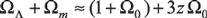(8)

It follows from (8) that for Ω0 = 0.03 as z → 0 the total density ΩΛ + Ωm → 1.03. This result is consistent with the WMAP cosmic microwave background data that produced a value of Ω m = 1.02 ± 0.02.26 (Note that they considered it is all due to matter.) However, it follows from (3) and (8) that the universe will always be open, Ωm < 1 as it expands. The value of the total density ΩΛ + Ωm begins very large but is always greater than unity and as the universe expands ΩΛ asymptotically decreases as it approaches unity. Therefore, the universe expands to become asymptotically spatially flat, i.e. ΩΛ + Ωm → 1.

Carmeli concluded from (1) that the universe was infinite and curved. Because the present value of Ωm < 1, the universe must be negatively curved and infinite. But this conclusion is not necessitated by the equations, since they describe an isotropic, not homogeneous, centro-symmetric matter distribution.

Different interpretations are applied by different commentators on this. The figure for total energy density ~1.02 from the WMAP data is in agreement with this analysis, even though the WMAP calculation is model-dependent, and the comparison may not be really valid. Both standard big-bangers and Carmeli would agree that spatially the universe is flat or nearly flat.

Image by NASA/WMAP Science Team
Figure 4. Spatial curvature for closed (Ω0 > 1), open (Ω0 < 1) and flat, or Euclidean, (Ω0 = 1) space

But since the cosmological constant is a property of space-time-velocity in the Carmeli cosmology the value of Ωm determines the state (open or closed) of the universe. Initially, adherents to FL cosmology had believed the universe was slightly closed and expanding towards a flat state, but the high-z supernovae and WMAP observations changed that. According to the Carmeli model, the data indicate that space is now slightly open but accelerating towards a spatially flat state. See figure 4 for a graphical definition of open, flat (Euclidean) and closed spatial curvature.

A creationist cosmological interpretation based on Carmeli’s model is a finite universe with spatial curvature that is essentially Euclidean. In the past this was not the case as the concentration of matter curved space, but all that took place in the first few days of the Creation Week. Since equation (1) indicates that the universe (actually space-velocity) passed through three phases, from closed to open through the momentary flat space-velocity, it necessitates a finite and bounded universe. How can a closed finite universe become an open infinite universe? Here the creationist finite and bounded universe makes sense.

The accelerating power of the universe is God himself. Therefore, He is behind the cosmological constant. It is not the result of dark energy, but God’s Almighty power as he gave impetus to the universe. The parameter appears in the standard FL inflationary cosmologies because they have to add it to account for the observed effect. Carmeli more correctly constructed his model without a need for this parameter by describing the mass/energy tensor such that as the universe expands, the vacuum of space itself relaxes. It can be understood that as the universe expands, the total density tends to a vacuum energy density ΩΛ of unity (since Ω0 tends to zero). This means a totally flat universe in a totally relaxed state. It is as if the fabric of space itself has relaxed like the relaxing of a coiled spring.

## Conclusion

The cosmological general relativity of Carmeli can explain the expansion of the accelerating universe without the need to resort to dark matter. By making a reasonable assumption about the dependence of matter density on redshift, it is shown that dark matter can be eliminated completely from the universe . As in past centuries, dark matter has been invoked to account for motions that could not be explained with the then-known laws of physics. General Relativity was applied to the motion of the planets to solve the riddle of the advance of the perihelion of Mercury.1 There still remains the alleged dark matter found in halos around spiral galaxies. That is outside the scope of this paper, but Milgrom’s MOND27 is a good empirical fit28 and Carmeli’s new equations of motion offer a solution there also.29

The modified field equations used by Carmeli describe a universe that would be expected from a reading of the Bible. That is, a galactocentric universe—the Milky Way galaxy being at the centre of the universe. The equations don’t explicitly involve a dark energy or a cosmological constant term, but they describe the present visible universe very well. They tell us the universe is accelerating and an extrapolation describes a state in the past where the universe was given a big push to expand out to its present locations. The cosmological constant, or dark energy, really describes a property of space-velocity. The big push was God, but through the agency of the fabric of space itself. He is the unseen force in the universe. God designed the original creation in a state such that it would naturally expand, relaxing the fabric of space itself like an uncoiling spring.

Posted on homepage: 28 September 2006